## Filters

Sort by :
Q
Engineering
128 Views   |

The total energy of a particle, executing simple harmonic motion is

• Option 1)whereis the displacement from the mean position.

• Option 2)whereis the displacement from the mean position.

• Option 3)

independent ofwhereis the displacement from the mean position.

• Option 4)whereis the displacement from the mean position.

Under simple harmonic motion, total energy Total energy is independent of . Total energy of s.u.w = i.e total energy is indepedent of x Option 1) where is the displacement from the mean position. Incorrect option Option 2)   where is the displacement from the mean position. Incorrect option Option 3) independent of  where is the displacement from the mean position. Correct...
Engineering
149 Views   |

In forced oscillation of a particle the amplitude is maximum for a frequencyof the force, while the energy is maximum for a frequencyof the force, then

• Option 1)• Option 2)• Option 3)when damping is small andwhen damping is large

• Option 4)(i) The amplitude is maximum at resonance (ii) The energy is maximum at resonance Option 1) This option is correct. Option 2) This option is incorrect. Option 3)   when damping is small and    when damping is large  This option is incorrect. Option 4) This option is incorrect.
Engineering
155 Views   |

A particle of mass  m  is attached to a spring (of spring constant k)  and has a natural angular frequency. An external force   F(t)   proportional tois applied to the oscillator . The time displacement of the oscillator will be proportional to

• Option 1)• Option 2)• Option 3)• Option 4)As we discussed in @7249 where         x is proportional to     Option 1) This answer is incorrect. Option 2) This answer is correct. Option 3) This answer is incorrect. Option 4) This answer is incorrect.
Engineering
129 Views   |

A person standing on the bank of a river observes that the angle of elevation of the top of a tree on the opposite bank of the river is and when he retires 40 meters away from the tree the angle of   elevation becomes . The breadth of the river is

• Option 1)

• Option 2)

• Option 3)

• Option 4)

As we learnt in  Height and Distances - The height or length of an object or the distance between two distant objects can be determined with the help of trigonometric ratios. -  Let OB represent bank, OA=height=h          Option 1) This option is incorrect Option 2) This option is incorrect Option 3) This option is correct Option 4) This option is incorrect
Engineering
109 Views   |

Let   be such that  .If    and

then the value of  is :

• Option 1)

• Option 2)

• Option 3)

• Option 4)

As we learnt in  Trigonometric Ratios of Submultiples of an Angle - - wherein This shows the formulae for half angles and their doubles.        -----------------(i) -------------------(ii) Squaring and adding,   Option 1) This is incorrect option Option 2) This is incorrect option Option 3) This is correct option Option 4) This is incorrect option
Engineering
109 Views   |

The domain of the function is

• Option 1)

• Option 2)

• Option 3)

• Option 4)

As we learnt in  Domains and Ranges of Inverse Trigonometric Functions - For  Domain  Range  -     For expression to be defined, Combining, we get  Option 1) This option is incorrect Option 2) This option is correct Option 3) This option is incorrect Option 4) This option is incorrect
Engineering
145 Views   |

Let be three non­-zero vectors such that no two of these are collinear. If the vector is collinear with is collinear with ( being some non-­zero scalar) then equals

• Option 1)

• Option 2)

• Option 3)

• Option 4)

As we learnt in  Collinear Vectors - Two vectors are said to be collinear if their directed line segments are parallel disregards to their direction.  - wherein If  and  are collinear , then  where K  R     is collinear with  Similarily  Eliminating  we get  Thus  Now Option 1) Incorrect Option 2) Incorrect Option 3) Incorrect Option 4) Correct
Engineering
112 Views   |

A particle is acted upon by constant forces which displace it from a point to the point . The work done in standard units by the forces is given by

• Option 1)

25

• Option 2)

30

• Option 3)

40

• Option 4)

15

As we learnt in  Applications of Vectors - - wherein Work done against a constant force  over a displacement      Work done by forces  Work done =  Option 1) 25 Incorrect Option 2) 30 Incorrect Option 3) 40 Correct Option 4) 15 Incorrect
Engineering
142 Views   |

If  are non-­coplanar vectors and is a real number, then the vectors are non-­coplanar for

• Option 1)

all except two values of

• Option 2)

all except one values of

• Option 3)

all values of

• Option 4)

no value of

As we learnt in  Coplanar vectors - - wherein ,  and  are three vectors.    Condition for given three vectors to be Coplanar is  They are non coplanar for all real  except  Option 1) all except two values of Correct Option 2) all except one values of Incorrect Option 3) all values of  Incorrect Option 4) no value of   Incorrect
Engineering
110 Views   |

If     is

• Option 1)

• Option 2)

• Option 3)

• Option 4)

As we learnt in  Differential Equations - An equation involving independent variable (x), dependent variable (y) and derivative of dependent variable with respect to independent variable  - wherein eg:          Option 1) This option is correct Option 2) This option is incorrect Option 3) This option is incorrect Option 4) This option is incorrect
Engineering
115 Views   |

The differential equation for the family of curves where is an arbitrary constant is

• Option 1)

• Option 2)

• Option 3)

• Option 4)

As we learnt in  Formation of Differential Equations - A differential equation can be derived from its equation by the process of differentiation and other algebraical process of elimination -       Option 1) Correct option Option 2) Incorrect option Option 3) Incorrect option Option 4) Incorrect option
Engineering
156 Views   |

The solution of the differential equation   is

• Option 1)

• Option 2)

• Option 3)

• Option 4)

As we learnt in  Bernoulli's Equation - - wherein     Divided by  Put    Option 1) Incorrect option Option 2) Correct option Option 3) Incorrect option Option 4) Incorrect option
Engineering
103 Views   |

A line with direction cosines proportional to 2, 1, 2 meets each of the lines   and The co­ordinates of each of the points of intersection are given by

• Option 1)

• Option 2)

• Option 3)

• Option 4)

As we learnt in  Direction Cosines - i)    ii)    If OP =r then the co-ordinates of P will be (lr,mr,nr) iii)    Direction cosines of X-axis are (1,0,0) iv)    Direction cosines of Y-axis are (0,1,0) v)    Direction cosines of Z-axis are (0,0,1) -     Cartesian eqution of a line - The equation of a line passing through two points and parallel to vector having direction ratios as is given by...
Engineering
99 Views   |

The intersection of the spheres and   s the same as the intersection of one of the sphere and the plane

• Option 1)

• Option 2)

• Option 3)

• Option 4)

As we learnt in  General equation (cartesian form ) - -    Intersection of spheres will give a  plane We get 2x-y-z=1 Option 1) Incorrect Option Option 2) Incorrect Option Option 3) Incorrect Option Option 4) Correct Option
Engineering
135 Views   |

Distance between two parallel planes is

• Option 1)

7/2

• Option 2)

5/2

• Option 3)

3/2

• Option 4)

9/2

As we learnt in  Distance of a point from plane (Cartesian form) - The length of perpendicular from to the plane is given by    -       Option 1) 7/2 Correct option  Option 2) 5/2 Incorrect option Option 3) 3/2 Incorrect option Option 4) 9/2 Incorrect option
Engineering
119 Views   |

A line makes the same angle , with each of the axis. If the angle , which it makes with -axis, is such that equals:

• Option 1)

3/5

• Option 2)

1/5

• Option 3)

2/3

• Option 4)

2/5

As we learnt in Direction Cosines - If are the angles which a vector makes with positive X-axis,Y-axis and Z-axis respectively then are known as diresction cosines, generally denoted by (l,m,n). - wherein     Given  Also,    Option 1) 3/5 Correct option Option 2) 1/5 Incorrect option Option 3) 2/3 Incorrect option Option 4) 2/5 Incorrect option
Engineering
118 Views   |

if  are G.P. then the value of the determinant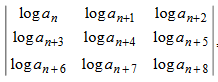is

• Option 1)

2

• Option 2)

1

• Option 3)

0

• Option 4)

-2

As we learnt in

Property of determinant -

If each element in a row ( or column ) of a determinant is written as the sum of two or more terms then the determinant can be written as the sum of two or more determinants

- wherein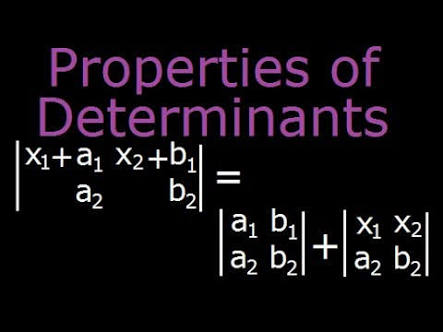Now

similarly

and so on.

Option 1)

2

Incorrect option

Option 2)

1

Incorrect option

Option 3)

0

Correct option

Option 4)

-2

Incorrect option

View More
Engineering
110 Views   |

Let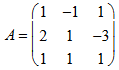and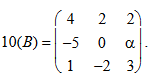If B is the inverse of matrix  , then is

• Option 1)

2

• Option 2)

-1

• Option 3)

-2

• Option 4)

5

As we learnt in  Inverse of a matrix -   -     and B is the inverse of A. So  Now  Option 1) 2 Incorrect answer Option 2) -1 Incorrect answer Option 3) -2 Incorrect answer Option 4) 5 Correct answer
Engineering
168 Views   |

Let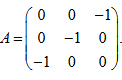.The only correct statement about the matrix A is

• Option 1)

does not exist

• Option 2)

is a unit matrix

• Option 3)

is a zero matrix

• Option 4)

As we learnt in

Multiplication of matrices -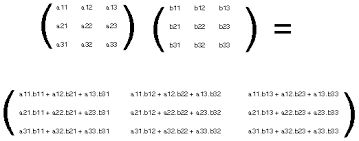-

Option 1)

does not exist

Incorrect option

Option 2)

is a unit matrix

Incorrect option

Option 3)

is a zero matrix

Incorrect option

Option 4)

Correct option

View More
Engineering
131 Views   |

If      then at least one root of the  equation

lies in the interval

• Option 1)

• Option 2)

• Option 3)

• Option 4)

As we learnt in  Rolle's Theorems - Let f(x) be a function of x subject to the following conditions. 1.  f(x) is continuous function of     2.  f'(x) is exists for every point :   3.   -    Let now and So having at least one root between (0,1) by Rollers Rule. Option 1) Incorrect option Option 2) Incorrect option Option 3) Correct option Option 4) Incorrect option
Exams
Articles
Questions